# Photon

Composition: Photon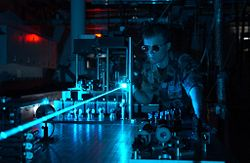Photons emitted in a coherent beam from a laser Elementary particle Boson Gauge boson Electromagnetic Albert Einstein (1905–1917) $\gamma \$or $\ h\nu$0 Stable 0 1

In modern physics, the photon is the elementary particle responsible for electromagnetic phenomena. It is the carrier of electromagnetic radiation of all wavelengths, including gamma rays, X-rays, ultraviolet light, visible light, infrared light, microwaves, and radio waves. It can also be considered a mediator for any type of electromagnetic interactions, including magnetic fields and electrostatic repulsion between like charges.

The photon differs from many other elementary particles, such as the electron and the quark, in that it has zero rest mass; therefore, it travels (in vacuum) at the speed of light (c). Photons are vital in our ability to see things around us, without their existence we would not be able to have a visual sense of our surroundings.

The photon concept has led to momentous advances in experimental and theoretical physics, such as lasers, Bose–Einstein condensation, quantum field theory, and the probabilistic interpretation of quantum mechanics. According to the Standard Model of particle physics, photons are responsible for producing all electric and magnetic fields, and are themselves the product of requiring that physical laws have a certain symmetry at every point in spacetime. The intrinsic properties of photons—such as charge, mass and spin—are determined by the properties of this gauge symmetry.

The concept of photons is applied to many areas such as photochemistry, high-resolution microscopy, and measurements of molecular distances. Recently, photons have been studied as elements of quantum computers and for sophisticated applications in optical communication such as quantum cryptography.

## Etymology and symbolism

The photon was originally called a “light quantum” (das Lichtquant) by Albert Einstein. The modern name photon derives from the Greek word for light, φῶς, (transliterated phôs), and was coined in 1926 by the physical chemist Gilbert N. Lewis, who published a speculative theory in which photons were “un-creatable and indestructible.” Although Lewis' theory was never accepted—being contradicted by many experiments—his new name, photon, was adopted immediately by most physicists.

In physics, a photon is usually denoted by the symbol $\gamma \!$, the Greek letter gamma. This symbol for the photon probably derives from gamma rays, which were discovered and named in 1900 by Paul Ulrich Villard and shown to be a form of electromagnetic radiation in 1914 by Rutherford and Andrade. In chemistry and optical engineering, photons are usually symbolized by $h\nu \!$, the energy of a photon, where $h\!$is Planck's constant and the Greek letter $\nu \!$(nu) is the photon's frequency. Much less commonly, the photon can be symbolized by hf, where its frequency is denoted by f.

## Dual properties of wave and particle

The photon is considered to have both wave and particle properties. As a wave, a single photon is distributed over space and shows wave-like phenomena, such as refraction by a lens and destructive interference when reflected waves cancel each other out; however, as a particle, it can only interact with matter by transferring the fixed amount (quantum) of energy "E," where:

$E={\frac {hc}{\lambda }},$where h is Planck's constant, c is the speed of light, and $\lambda$is the photon's wavelength. This is different from a classical wave, which may gain or lose arbitrary amounts of energy.

For visible light, the energy carried by a single photon would be very tiny—approximately 4 x 10−19 joules. This energy is just sufficient to excite a single molecule in a photoreceptor cell of an eye, thus contributing to vision.

Apart from energy a photon also carries momentum and has a polarization. It follows the laws of quantum mechanics, which means that often these properties do not have a well-defined value for a given photon. Rather, they are defined as a probability to measure a certain polarization, position, or momentum. For example, although a photon can excite a single molecule, it is often impossible to predict beforehand which molecule will be excited.

## Historical development

Main article: Light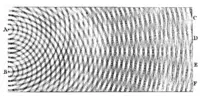Thomas Young's double-slit experiment in 1805 showed that light can act as a wave, helping to defeat early particle theories of light.

In most theories up to the eighteenth century, light was pictured as being made up of particles. Since particle models cannot easily account for the refraction and diffraction of light, wave theories of light were proposed by René Descartes (1637), Robert Hooke, (1665), and Christian Huygens (1678); however, particle models remained dominant, chiefly due to the influence of Isaac Newton. In the early nineteenth century, Thomas Young and August Fresnel clearly demonstrated the interference and diffraction of light and by 1850 wave models were generally accepted. In 1865, James Clerk Maxwell's prediction that light was an electromagnetic wave—which was confirmed experimentally in 1888 by Heinrich Hertz's detection of radio waves—seemed to be the final blow to particle models of light.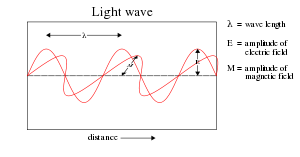In 1900, Maxwell's theoretical model of light as oscillating electric and magnetic fields seemed complete. However, several observations could not be explained by any wave model of electromagnetic radiation, leading to the idea that light-energy was packaged into quanta described by E=hν. Later experiments showed that these light-quanta also carry momentum and, thus, can be considered particles: the photon concept was born, leading to a deeper understanding of the electric and magnetic fields themselves.

The Maxwell wave theory, however, does not account for all properties of light. The Maxwell theory predicts that the energy of a light wave depends only on its intensity, not on its frequency; nevertheless, several independent types of experiments show that the energy imparted by light to atoms depends only on the light's frequency, not on its intensity. For example, some chemical reactions are provoked only by light of frequency higher than a certain threshold; light of frequency lower than the threshold, no matter how intense, does not initiate the reaction. Similarly, electrons can be ejected from a metal plate by shining light of sufficiently high frequency on it (the photoelectric effect); the energy of the ejected electron is related only to the light's frequency, not to its intensity.

At the same time, investigations of blackbody radiation carried out over four decades (1860–1900) by various researchers culminated in Max Planck's hypothesis that the energy of any system that absorbs or emits electromagnetic radiation of frequency $\nu$is an integer multiple of an energy quantum $E=h\nu$. As shown by Albert Einstein, (German) A. Einstein, (1909). "Über die Entwicklung unserer Anschauungen über das Wesen und die Konstitution der Strahlung (trans.) The Development of Our Views on the Composition and Essence of Radiation)". Physikalische Zeitschrift 10: 817–825. (German).</ref> some form of energy quantization must be assumed to account for the thermal equilibrium observed between matter and electromagnetic radiation.

Since the Maxwell theory of light allows for all possible energies of electromagnetic radiation, most physicists assumed initially that the energy quantization resulted from some unknown constraint on the matter that absorbs or emits the radiation.

The modern concept of the photon was developed gradually (1905–1917) by Albert Einstein to explain experimental observations that did not fit the classical wave model of light. In particular, the photon model accounted for the frequency dependence of light's energy, and explained the ability of matter and radiation to be in thermal equilibrium.

Other physicists sought to explain these anomalous observations by semiclassical models, in which light is still described by Maxwell's equations, but the material objects that emit and absorb light are quantized. Although these semiclassical models contributed to the development of quantum mechanics, further experiments proved Einstein's hypothesis that light itself is quantized; the quanta of light are photons.

In 1905, Einstein proposed that energy quantization was a property of electromagnetic radiation itself. Although he accepted the validity of Maxwell's theory, Einstein pointed out that many anomalous experiments could be explained if the energy of a Maxwellian light wave were localized into point-like quanta that move independently of one another, even if the wave itself is spread continuously over space. In 1909 and 1916, Einstein showed that, if Planck's law of black-body radiation is accepted, the energy quanta must also carry momentum $p=h/\lambda$, making them full-fledged particles. This photon momentum was observed experimentally by Arthur Compton, for which he received the Nobel Prize in 1927. The pivotal question was then: how to unify Maxwell's wave theory of light with its experimentally observed particle nature? The answer to this question occupied Albert Einstein for the rest of his life, and was solved in quantum electrodynamics and its successor, the Standard Model.

### Early objections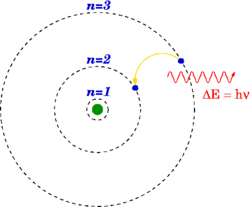Up to 1923, most physicists were reluctant to accept that electromagnetic radiation itself was quantized. Instead, they tried to account for photon behavior by quantizing matter, as in the Bohr model of the hydrogen atom (shown here). Although all semiclassical models have been disproved by experiment, these early atomic models led to quantum mechanics.

Einstein's 1905 predictions were verified experimentally in several ways within the first two decades of the twentieth century, as recounted in Robert Millikan's Nobel lecture. However, before Compton's experiment showing that photons carried momentum proportional to their frequency (1922), most physicists were reluctant to believe that electromagnetic radiation itself might be particulate. (This reluctance is evident in the Nobel lectures of Wien, Planck and Millikan.) This reluctance was understandable, given the success and plausibility of Maxwell's electromagnetic wave model of light. Therefore, most physicists assumed rather that energy quantization resulted from some unknown constraint on the matter that absorbs or emits radiation. Niels Bohr, Arnold Sommerfeld and others developed atomic models with discrete energy levels that could account qualitatively for the sharp spectral lines and energy quantization observed in the emission and absorption of light by atoms; their models agreed excellently with the spectrum of hydrogen, but not with those of other atoms. It was only the Compton scattering of a photon by a free electron (which can have no energy levels, since it has no internal structure) that convinced most physicists that light itself was quantized.

Even after Compton's experiment, Bohr, Hendrik Kramers and John Slater made one last attempt to preserve the Maxwellian continuous electromagnetic field model of light, the so-called BKS model. To account for the then-available data, two drastic hypotheses had to be made:

• Energy and momentum are conserved only on the average in interactions between matter and radiation, not in elementary processes such as absorption and emission. This allows one to reconcile the discontinuously changing energy of the atom (jump between energy states) with the continuous release of energy into radiation.
• Causality is abandoned. For example, spontaneous emissions are merely emissions induced by a "virtual" electromagnetic field.

However, refined Compton experiments showed that energy-momentum is conserved extraordinarily well in elementary processes; and also that the jolting of the electron and the generation of a new photon in Compton scattering obey causality to within 10 ps. Accordingly, Bohr and his co-workers gave their model “as honorable a funeral as possible“. Nevertheless, the BKS model inspired Werner Heisenberg in his development of quantum mechanics.

A few physicists persisted in developing semiclassical models in which electromagnetic radiation is not quantized, but matter obeys the laws of quantum mechanics. Although the evidence for photons from chemical and physical experiments was overwhelming by the 1970s, this evidence could not be considered as absolutely definitive; since it relied on the interaction of light with matter, a sufficiently complicated theory of matter could in principle account for the evidence. Nevertheless, all semiclassical theories were refuted definitively in the 1970s and 1980s by elegant photon-correlation experiments. Hence, Einstein's hypothesis that quantization is a property of light itself is considered to be proven.

## Physical properties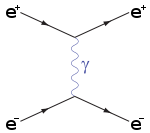A Feynman diagram of the exchange of a virtual photon (symbolized by a wavy-line and a gamma, $\gamma \,$) between a positron and an electron.

The basic photon is massless, has no electric charge and does not decay spontaneously in empty space. A photon has two possible polarization states and is described by exactly three continuous parameters: the components of its wave vector, which determine its wavelength $\lambda \!$and its direction of propagation. The photon is the gauge boson for electromagnetic interaction (they are responsible for electromagnetic interactions).

Photons are emitted in many natural processes, e.g., when a charge is accelerated, during a chemical reaction, electron transition to a lower energy level, or when a particle and its antiparticle are annihilated. Photons are absorbed in the reversed processes which correspond to those mentioned above: for example in an electron transitions to a higher energy level.

In empty space, the photon moves at $c\!$(the speed of light) and its energy $E\!$and momentum $\mathbf {p}$are related by $E=c\,p\!$, where $p\!$is the magnitude of the momentum. For comparison, the corresponding equation for particles with a mass $m\!$would be $E^{2}=c^{2}p^{2}+m^{2}c^{4}\!$, as shown in special relativity.

The energy and momentum of a photon depend only on its frequency $\nu \!$or, equivalently, its wavelength $\lambda \!$$E=\hbar \omega =h\nu ={\frac {hc}{\lambda }}$$\mathbf {p} =\hbar \mathbf {k}$and consequently the magnitude of the momentum is

$p=\hbar k={\frac {h}{\lambda }}={\frac {h\nu }{c}}$where $\hbar =h/2\pi \!$(known as Dirac's constant or Planck's reduced constant);

$\mathbf {k}$is the wave vector (with the wave number)

$k=2\pi /\lambda \!$as its magnitude) and

$\omega =2\pi \nu \!$is the angular frequency.

The photon also carries spin angular momentum that does not depend on its frequency. The magnitude of its spin is ${\sqrt {2}}\hbar$and the component measured along its direction of motion.

To illustrate the significance of these formulae, the annihilation of a particle with its antiparticle must result in the creation of at least two photons for the following reason. In the center of mass frame, the colliding antiparticles have no net momentum, whereas a single photon always has momentum. Hence, conservation of momentum requires that at least two photons are created, with zero net momentum. The energy of the two photons—or, equivalently, their frequency—may be determined from conservation of momentum.

The classical formulae for the energy and momentum of electromagnetic radiation can be re-expressed in terms of photon events. For example, the pressure of electromagnetic radiation on an object derives from the transfer of photon momentum per unit time and unit area to that object, since pressure is force per unit area and force is the change in momentum per unit time. The idea of the solar sail comes from this concept.

## Wave–particle duality and uncertainty principles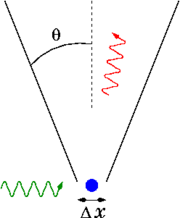Heisenberg's thought experiment for locating an electron (shown in blue) with a high-resolution gamma-ray microscope. The incoming gamma ray (shown in green) is scattered by the electron up into the microscope's aperture angle θ. The scattered gamma ray is shown in red. Classical optics shows that the electron position can be resolved only up to an uncertainty Δx that depends on θ and the wavelength λ of the incoming light.

Photons, like all quantum objects, exhibit both wave-like and particle-like properties. Their dual wave–particle nature can be difficult to visualize. The photon displays clearly wave-like phenomena such as diffraction and interference on the length scale of its wavelength. For example, a single photon passing through a double-slit experiment lands on the screen with a probability distribution given by its interference pattern determined by Maxwell's equations. However, experiments confirm that the photon is not a short pulse of electromagnetic radiation; it does not spread out as it propagates, nor does it divide when it encounters a beam splitter. Rather, the photon seems like a point-like particle, since it is absorbed or emitted as a whole by arbitrarily small systems, systems much smaller than its wavelength, such as an atomic nucleus (≈10–15 m across) or even the point-like electron. Nevertheless, the photon is not a point-like particle whose trajectory is shaped probabilistically by the electromagnetic field, as conceived by Einstein and others; that hypothesis was also refuted by the photon-correlation experiments cited above. According to our present understanding, the electromagnetic field itself is produced by photons, which in turn result from a local gauge symmetry and the laws of quantum field theory

A key element of quantum mechanics is Heisenberg's uncertainty principle, which forbids the simultaneous measurement of the position and momentum of a particle along the same direction. Remarkably, the uncertainty principle for charged, material particles requires the quantization of light into photons, and even the frequency dependence of the photon's energy and momentum. An elegant illustration is Heisenberg's thought experiment for locating an electron with an ideal microscope.

Both photons and material particles such as electrons create analogous interference patterns when passing through a double-slit experiment. For photons, this corresponds to the interference of a Maxwell light wave whereas, for material particles, this corresponds to the interference of the Schrödinger wave equation. Although this similarity might suggest that Maxwell's equations are simply Schrödinger's equation for photons, most physicists do not agree. For one thing, they are mathematically different; most obviously, Schrödinger's one equation solves for a complex field, whereas Maxwell's four equations solve for real fields. More generally, the normal concept of a Schrödinger probability wave function cannot be applied to photons. Being massless, they cannot be localized without being destroyed; technically, photons cannot have a position eigenstate $|\mathbf {r} \rangle$, and, thus, the normal Heisenberg uncertainty principle does not pertain to photons.

## Contributions to the mass of a system

The energy of a system that emits a photon is decreased by the energy $E$of the photon as measured in the rest frame of the emitting system, which may result in a reduction in mass in the amount ${E}/{c^{2}}$. Similarly, the mass of a system that absorbs a photon is increased by a corresponding amount.

Since photons contribute to the stress-energy tensor, they exert a gravitational attraction on other objects, according to the theory of general relativity. Conversely, photons are themselves affected by gravity; their normally straight trajectories may be bent by warped spacetime, as in gravitational lensing, and their frequencies may be lowered by moving to a higher gravitational potential, as in the Pound-Rebka experiment. However, these effects are not specific to photons; exactly the same effects would be predicted for classical electromagnetic waves.

## Photons in matter

Light that travels through transparent matter does so at a lower speed than c, the speed of light in a vacuum. For example, photons suffer so many collisions on the way from the core of the sun that radiant energy can take years to reach the surface; however, once in open space, a photon only takes 8.3 minutes to reach Earth. The factor by which the speed is decreased is called the refractive index of the material. In a classical wave picture, the slowing can be explained by the light inducing electric polarization in the matter, the polarized matter radiating new light, and the new light interfering with the original light wave to form a delayed wave. In a particle picture, the slowing can instead be described as a blending of the photon with quantum excitations of the matter (quasi-particles such as phonons and excitons) to form a polariton; this polariton has a nonzero effective mass, which means that it cannot travel at the speed of light. Light of different frequencies may travel through matter at different speeds; this is called dispersion.

where, as above, $E$and $p$are the polariton's energy and momentum magnitude, and $\omega$and $k$are its angular frequency and wave number, respectively. In some cases, the dispersion can result in extremely slow speeds of light in matter.

Photons can also be absorbed by nuclei, atoms or molecules, provoking transitions between their energy levels. A classic example is the molecular transition of retinal (C20H28O), which is responsible for vision, as discovered in 1958 by Nobel laureate biochemist George Wald and co-workers. As shown here, the absorption provokes a cis-trans isomerization that, in combination with other such transitions, is transduced into nerve impulses. The absorption of photons can even break chemical bonds, as in the photodissociation of chlorine.

## Technological applications

Photons have many applications in technology. These examples are chosen to illustrate applications of photons per se, rather than general optical devices such as lenses, that could operate under a classical theory of light.

A Laser is a device that emits light through a specific mechanism. A typical laser emits light in a narrow, low-divergence beam and with a well-defined wavelength (corresponding to a particular color if the laser is operating in the visible spectrum). This is in contrast to a light source such as the incandescent light bulb, which emits into a large solid angle and over a wide spectrum of wavelength. Lasers have become ubiquitous, finding utility in thousands of highly varied applications in every section of modern society, including consumer electronics, information technology, science, medicine, industry, law enforcement, entertainment, and the military.

Planck's energy formula $E=h\nu$is often used by engineers and chemists in design, both to compute the change in energy resulting from a photon absorption and to predict the frequency of the light emitted for a given energy transition. For example, the emission spectrum of a fluorescent light bulb can be designed using gas molecules with different electronic energy levels and adjusting the typical energy with which an electron hits the gas molecules within the bulb.

Under some conditions, an energy transition can be excited by two photons that individually would be insufficient. This allows for higher resolution microscopy, because the sample absorbs energy only in the region where two beams of different colors overlap significantly, which can be made much smaller than the excitation volume of a single beam. Moreover, these photons cause less damage to the sample, since they are of lower energy.

In some cases, two energy transitions can be coupled so that, as one system absorbs a photon, another nearby system "steals" its energy and re-emits a photon of a different frequency. This is the basis of fluorescence resonance energy transfer, which is used to measure molecular distances.

Photons are essential in some aspects of optical communication such as fiber optic cables. Light propagates through the fiber with little attenuation compared to electrical cables. This allows long distances to be spanned with few repeaters.

### Detection of photons

Individual photons can be detected by several methods. The classic photomultiplier tube exploits the photoelectric effect; a photon landing on a metal plate ejects an electron, initiating an ever-amplifying avalanche of electrons. Charge-coupled device chips use a similar effect in semiconductors; an incident photon generates a charge on a microscopic capacitor that can be detected. Other detectors such as Geiger counters use the ability of photons to ionize gas molecules, causing a detectable change in conductivity.